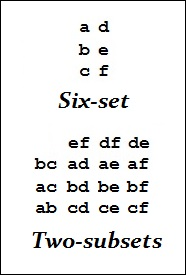# Log24

## Monday, September 19, 2016

### Squaring the Pentagon

Filed under: General,Geometry — m759 @ 10:00 AM

The "points" and "lines" of finite  geometry are abstract
entities satisfying only whatever incidence requirements
yield non-contradictory and interesting results. In finite
geometry, neither the points nor the lines are required to
lie within any Euclidean (or, for that matter, non-Euclidean)
space.

Models  of finite geometries may, however, embed the
points and lines within non -finite geometries in order
to aid visualization.

For instance, the 15 points and 35 lines of PG(3,2) may
be represented by subsets of a 4×4 array of dots, or squares,
located in the Euclidean plane. These "lines" are usually finite
subsets of dots or squares and not*  lines of the Euclidean plane.

Example — See "4×4" in this journal.

Some impose on configurations from finite geometry
the rather artificial requirement that both  points and lines
must be representable as those of a Euclidean plane.

Example:  A Cremona-Richmond pentagon —

A square version of these 15 "points" —A 1905 square version of these 15 "points"
with digits instead of letters —

See Parametrizing the 4×4 Array
(Log24 post of Sept. 13, 2016).

Update of 8 AM ET Sunday, Sept. 25, 2016 —
For more illustrations, do a Google image search
on "the 2-subsets of a 6-set." (See one such search.)

* But in some models are subsets of the grid lines
that separate squares within an array.